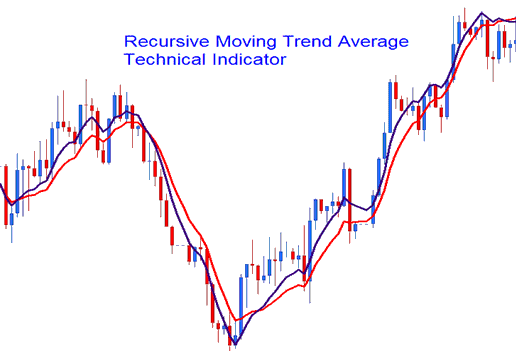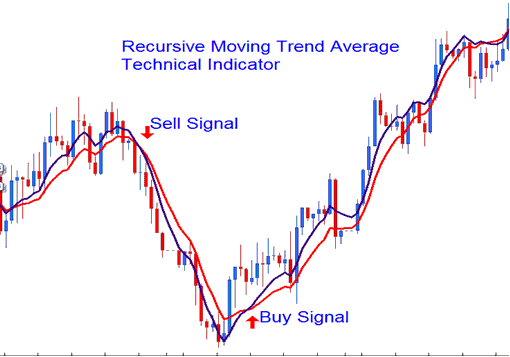# Recursive Moving Trend Average  Forex Technical Analysis and Forex Trading Signals

This Indicator is calculated using a mathematical polynomial fit, the formula is referred as the Recursive Moving Polynomial Fit.

This formula used to calculate this indicator only requires a small set of previous data to calculate and predict the next direction of price movement. The example below shows two Recursive Averages combined to form a crossover trading system method.## Forex Technical Analysis and Generating Forex Trading Signals

The best technical analysis method is the crossover method where you can combine two recursive  averages, such as the 14 and 21. When the two crossovers each other upwards then that is a bullish signal while a downward crossover is a bearish signal.The Recursive Average looks similar to the traditional moving average, the only difference is that is much smoother due to the method of calculation that it uses and much less prone to whipsaws.

Limited Time 100% Account Opening Bonus - Open Account

Top 100 Forex Broker Rankings